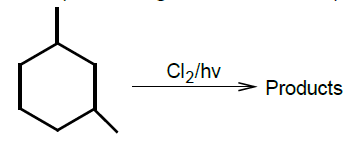The monochlorinated products (excluding stereo-isomers) obtained from the reaction is:(1) 4            (2) 5              (3) 6            (4) 7

Concept Questions :-

Alkanes, Alkenes and Alkynes - Chemical Properties
High Yielding Test Series + Question Bank - NEET 2020

Difficulty Level:

3-hexyne can be converted to trans-3-hexene by the action of:

1. ${\mathrm{H}}_{2}$ - Pd/ ${\mathrm{BaSO}}_{4}$                       2. Li-Liq. ${\mathrm{NH}}_{3}$

3. ${\mathrm{H}}_{2}$ - Pt ${\mathrm{O}}_{2}$                                4. ${\mathrm{NaBH}}_{4}$

Concept Questions :-

Alkanes, Alkenes and Alkynes -Methods of Preparation
High Yielding Test Series + Question Bank - NEET 2020

Difficulty Level:

Which one of the following has the shortest carbon-carbon bond length?

1. Benzene                                  2. Ethene

3. Ethyne                                    4. Ethane

Concept Questions :-

Aliphatic Hydrocarbon- physical properties
High Yielding Test Series + Question Bank - NEET 2020

Difficulty Level:

Reaction of HBr with propene in the presence of peroxide gives

1. iso-propyl bromide

2. 3-bromo propane

3. allyl bromide

4. n-propyl bromide

High Yielding Test Series + Question Bank - NEET 2020

Difficulty Level:

In Friedel-Craft's synthesis of toluene, the reactants in addition to anhydrous ${\mathrm{AlCl}}_{3}$ are

(a)

(b) ${\mathrm{C}}_{6}{\mathrm{H}}_{5}\mathrm{Cl}$ + ${\mathrm{CH}}_{3}\mathrm{Cl}$

(c) ${\mathrm{C}}_{6}{\mathrm{H}}_{6}$ + ${\mathrm{CH}}_{4}$

(d) ${\mathrm{C}}_{6}{\mathrm{H}}_{6}$ + ${\mathrm{CH}}_{3}\mathrm{Cl}$

Concept Questions :-

Aromatic Hydrocarbons - Benzene - Structure, Preparation & Chemical Reactions
High Yielding Test Series + Question Bank - NEET 2020

Difficulty Level:

The reaction of toluene with ${\mathrm{Cl}}_{2}$ in the presence of ${\mathrm{FeCl}}_{3}$ gives 'X' and reaction in presence of light gives 'Y'. Thus, 'X' and  'Y' are

1. X = benzal chloride, Y= o-chlorotoluene

2. X = m-chlorotoluene, Y= p-chlorotoluene

3. X = o and p-chlorotoluene, Y = trichloromethyl benzene

4. X = benzal chloride, Y = m-chlorotoluene

Concept Questions :-

Aromatic Hydrocarbons - Functional Groups, Directive Influence in Monosubstituted Benzene
High Yielding Test Series + Question Bank - NEET 2020

Difficulty Level:

In the reaction, ${\mathrm{C}}_{6}{\mathrm{H}}_{5}{\mathrm{CH}}_{3}\stackrel{\mathrm{Oxidation}}{\to }$$\stackrel{\mathrm{NaOH}}{\to }$B

the product C is:

1. ${\mathrm{C}}_{6}{\mathrm{H}}_{5}\mathrm{OH}$                              2. ${\mathrm{C}}_{6}{\mathrm{H}}_{6}$

3. ${\mathrm{C}}_{6}{\mathrm{H}}_{5}\mathrm{COONa}$                        4. ${\mathrm{C}}_{6}{\mathrm{H}}_{5}\mathrm{ONa}$

Concept Questions :-

Aromatic Hydrocarbons - Benzene - Structure, Preparation & Chemical Reactions
High Yielding Test Series + Question Bank - NEET 2020

Difficulty Level:

When acetylene is passed through dil. ${\mathrm{H}}_{2}{\mathrm{SO}}_{4}$ in presence of ${\mathrm{HgSO}}_{4}$, the compound formed is

1. ether                                    2. ketone

3. acetic acid                             4. acetaldehyde

Concept Questions :-

Alkanes, Alkenes and Alkynes - Chemical Properties
High Yielding Test Series + Question Bank - NEET 2020

Difficulty Level:

Acetylenic hydrogens are acidic because

1. sigma electron density of C-H bond in acetylene is nearer to carbon which has 50% s-character

2. acetylene has only one hydrogen on each carbon

3. acetylene contains least number of hydrogens among the possible hydrocarbons having two carbons

4. acetylene belong to the class of alkynes with molecular formula ${\mathrm{C}}_{\mathrm{n}}{\mathrm{H}}_{2\mathrm{n}-2}$

Concept Questions :-

Alkanes, Alkenes and Alkynes - Chemical Properties
High Yielding Test Series + Question Bank - NEET 2020

Difficulty Level:

A compound is treated with ${\mathrm{NaNH}}_{2}$ to give sodium salt. Identify the compound.

(a) ${\mathrm{C}}_{2}{\mathrm{H}}_{2}$                                 (b) ${\mathrm{C}}_{6}{\mathrm{H}}_{6}$

(c) ${\mathrm{C}}_{2}{\mathrm{H}}_{6}$                                 (d) ${\mathrm{C}}_{2}{\mathrm{H}}_{4}$

Concept Questions :-

Alkanes, Alkenes and Alkynes - Chemical Properties